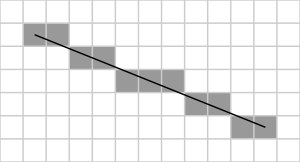# Implementing Bresenham’s Line Algorithm in C++

Bresenham’s line algorithm is an algorithm that determines the points of an n-dimensional raster that should be selected in order to form a close approximation to a straight line between two points. It is commonly used to draw lines on a computer screen, as it uses only integer addition, subtraction and bit shifting.Illustration of the result of Bresenham’s line algorithm. (0,0) is at the top left corner of the grid, (1,1) is at the top left end of the line and (11, 5) is at the bottom right end of the line.

(Via Wikipedia)

Here is C++ implementation of this algorithm.

```#include <graphics.h>
#include <stdlib.h>
#include <stdio.h>
#include <conio.h>
#include <iostream.h>
int main(void)
{
/* request auto detection */
int gdriver = DETECT, gmode, errorcode;

/* initialize graphics and local variables */
initgraph(&gdriver, &gmode, "C:\\tc\\bgi");

cout<<"\n Enter X1,Y1,X2,Y2";
int x1,y1,x2,y2;
cin>>x1>>y1>>x2>>y2;

int dx=x2-x1;
int dy=y2-y1;
int x=x1;
int y=y1;

int e=(2*dy)-dx;
for (int i=0;i<=dx;i++)
{
putpixel(x,y,15);
while(e>=0)
{
y=y+1;
e=e-(2*dx);
}
x=x+1;
e=e+(2*dy);
}

/* clean up */
getch();
closegraph();
return 0;
}
```

Output: